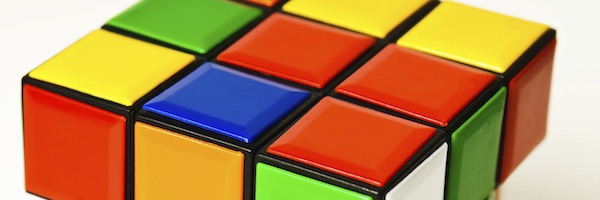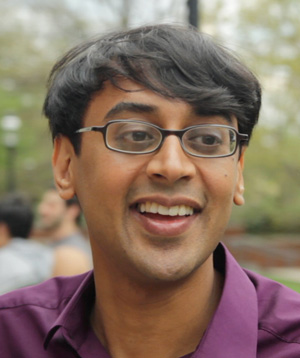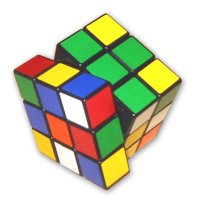# Numbers, toys and musicManjul Bhargava is a mathematician who in 2014 won one of the most important prizes in mathematics, the famous Fields Medal. His idea of mathematics is interesting. "I think that the reasons for doing maths are similar to those for doing music or art," he says. "It's about contributing to a certain understanding of the world and ourselves." Bhargava has been described as having "extraordinary creativity".  He works in number theory, the study of the whole numbers. This area has fascinated mathematicians for thousands of years and is famous for problems that are fiendishly difficult to solve.##### Manjul Bhargava

Bhargava believes that one of the keys to solving hard problems is to look at them in a new way. One of his first exciting moments of discovery came when he was graduate student. One night, at around midnight, he was thinking about a problem inspired by the famous German mathematician Carl Friedrich Gauss (1777-1855).

Gauss thought about numbers that could be expressed as sums of square numbers, such as 4, 9, 16 and so on. In 1770 the mathematician Joseph-Louis Lagrange had shown that every natural number can be written as such a sum: $$0 = 0^2+0^2+0^2+0^2, \\ 1 = 1^2+0^2+0^2+0^2, \\ 2 = 1^2+1^2+0^2+0^2, \\ 3 = 1^2+1^2+1^2+0^2, \\ \vdots \\ 30=1^2+2^2+3^2+4^2, \\ 31 = 1^2+1^2+2^2+5^2, \\$$ and so on. (To see why you need four squares, try writing 7 using just three.)

Sums of squares are an example of more complicated sums which are called quadratic forms (you will learn about quadratic forms later on at school). Quadratic forms lie at the heart of many interesting questions in number theory, and in his book Gauss gave a way of combining two quadratic forms to obtain a third – called Gauss composition. This has become an important mathematical tool in the area.##### Playing with mathematics. Image from Wikipedia.

Bhargava was amazed when he first read Gauss' proof of this composition law, which ran to over 20 pages. But he thought that there had to be an easier way. "I was in my dorm room getting ready to go to bed. I had these Rubik's cubes in my room and I was just looking at one," says Bhargava. "I have this memory of thinking, 'What happens if you put numbers on the corners of this Rubik's cube? Instead of just thinking of it as a simple cube, think of it with numbers on the corners.' I put numbers on the corners of that cube and I did some manipulations, and I saw three quadratic forms coming out. I decided not to go to sleep, that I'd figure out what these three quadratic expressions [were], how were they related."

Instead of the very difficult mathematics he had read in Gauss, Bhargava's new way of looking at Gauss' composition could be expressed in just a few lines. Moreover, it allowed Bhargava to take the maths even further: "This opened the door to twelve more analogs of Gauss' composition – but the first was opened in part due to playing with toys."

As this example shows, an important part of Bhargava's work is finding new ways to think about established mathematics: "If you think about things the way someone else does then you will never understand it as well as if you think about it your own way," he explains. "The best way to get people to think about it their own way, students in particular, is for them to discover it themselves, or to discover it in the most bare bones way. Play around with it, experiment and then come up with the results yourself. Develop your own way of thinking about those sequences of steps, and then you'll also understand why those sequences of steps are relevant. Maybe you'll develop your own sequence of steps and then of course you'll know why you're doing it that way."

For Bhargava, it is this creative process that leads to progress and also the reason he enjoys his work. "For me, when I had that new way of thinking about the Gauss composition, that was the moment when I really, truly enjoyed understanding what's going on," he recalls. "Because that's when I really did truly understand. I could have regurgitated exactly what Gauss did, or regurgitated exactly how modern mathematicians reformulated everything. When I had my own way of thinking about it, that's when I really felt: 'Now I understand, this is really fun, this is really great.' "